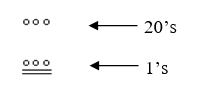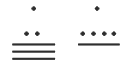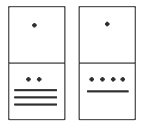$$\newcommand{\id}{\mathrm{id}}$$ $$\newcommand{\Span}{\mathrm{span}}$$ $$\newcommand{\kernel}{\mathrm{null}\,}$$ $$\newcommand{\range}{\mathrm{range}\,}$$ $$\newcommand{\RealPart}{\mathrm{Re}}$$ $$\newcommand{\ImaginaryPart}{\mathrm{Im}}$$ $$\newcommand{\Argument}{\mathrm{Arg}}$$ $$\newcommand{\norm}{\| #1 \|}$$ $$\newcommand{\inner}{\langle #1, #2 \rangle}$$ $$\newcommand{\Span}{\mathrm{span}}$$

# 14.5: The Mayan Numeral System

$$\newcommand{\vecs}{\overset { \rightharpoonup} {\mathbf{#1}} }$$ $$\newcommand{\vecd}{\overset{-\!-\!\rightharpoonup}{\vphantom{a}\smash {#1}}}$$$$\newcommand{\id}{\mathrm{id}}$$ $$\newcommand{\Span}{\mathrm{span}}$$ $$\newcommand{\kernel}{\mathrm{null}\,}$$ $$\newcommand{\range}{\mathrm{range}\,}$$ $$\newcommand{\RealPart}{\mathrm{Re}}$$ $$\newcommand{\ImaginaryPart}{\mathrm{Im}}$$ $$\newcommand{\Argument}{\mathrm{Arg}}$$ $$\newcommand{\norm}{\| #1 \|}$$ $$\newcommand{\inner}{\langle #1, #2 \rangle}$$ $$\newcommand{\Span}{\mathrm{span}}$$ $$\newcommand{\id}{\mathrm{id}}$$ $$\newcommand{\Span}{\mathrm{span}}$$ $$\newcommand{\kernel}{\mathrm{null}\,}$$ $$\newcommand{\range}{\mathrm{range}\,}$$ $$\newcommand{\RealPart}{\mathrm{Re}}$$ $$\newcommand{\ImaginaryPart}{\mathrm{Im}}$$ $$\newcommand{\Argument}{\mathrm{Arg}}$$ $$\newcommand{\norm}{\| #1 \|}$$ $$\newcommand{\inner}{\langle #1, #2 \rangle}$$ $$\newcommand{\Span}{\mathrm{span}}$$

## Background

As you might imagine, the development of a base system is an important step in making the counting process more efficient. Our own base-ten system probably arose from the fact that we have 10 fingers (including thumbs) on two hands. This is a natural development. However, other civilizations have had a variety of bases other than ten. For example, the Natives of Queensland used a base-two system, counting as follows: “one, two, two and one, two two’s, much.” Some Modern South American Tribes have a base-five system counting in this way: “one, two, three, four, hand, hand and one, hand and two,” and so on. The Babylonians used a base-sixty (sexigesimal) system. In this chapter, we wrap up with a specific example of a civilization that actually used a base system other than 10.The Mayan civilization is generally dated from 1500 B.C.E to 1700 C.E. The Yucatan Peninsula (see map[i]) in Mexico was the scene for the development of one of the most advanced civilizations of the ancient world. The Mayans had a sophisticated ritual system that was overseen by a priestly class. This class of priests developed a philosophy with time as divine and eternal.[ii] The calendar, and calculations related to it, were thus very important to the ritual life of the priestly class, and hence the Mayan people. In fact, much of what we know about this culture comes from their calendar records and astronomy data. Another important source of information on the Mayans is the writings of Father Diego de Landa, who went to Mexico as a missionary in 1549.There were two numeral systems developed by the Mayans - one for the common people and one for the priests. Not only did these two systems use different symbols, they also used different base systems. For the priests, the number system was governed by ritual. The days of the year were thought to be gods, so the formal symbols for the days were decorated heads,[iii] like the sample to the left[iv] Since the basic calendar was based on 360 days, the priestly numeral system used a mixed base system employing multiples of 20 and 360. This makes for a confusing system, the details of which we will skip.

## The Mayan Number System

Instead, we will focus on the numeration system of the “common” people, which used a more consistent base system. As we stated earlier, the Mayans used a base-20 system, called the “vigesimal” system. Like our system, it is positional, meaning that the position of a numeric symbol indicates its place value. In the following table you can see the place value in its vertical format.[v]$$\begin{array}{|c|c|l|} \hline \text { Powers } & \text { Base-Ten Value } & \text { Place Name } \\ \hline 20^{7} & 12,800,000,000 & \text { Hablat } \\ \hline 20^{6} & 64,000,000 & \text { Alau } \\ \hline 20^{5} & 3,200,000 & \text { Kinchil } \\ \hline 20^{4} & 160,000 & \text { Cabal } \\ \hline 20^{3} & 8,000 & \text { Pic } \\ \hline 20^{2} & 400 & \text {Bak} \\ \hline 20^{1} & 20 & \text { Kal } \\ \hline 20^{0} & 1 & \text { Hun } \\ \hline \end{array}$$

In order to write numbers down, there were only three symbols needed in this system. A horizontal bar represented the quantity 5, a dot represented the quantity 1, and a special symbol (thought to be a shell) represented zero. The Mayan system may have been the first to make use of zero as a placeholder/number. The first 20 numbers are shown in the table to the right.[vi]

Unlike our system, where the ones place starts on the right and then moves to the left, the Mayan systems places the ones on the bottom of a vertical orientation and moves up as the place value increases.

When numbers are written in vertical form, there should never be more than four dots in a single place. When writing Mayan numbers, every group of five dots becomes one bar. Also, there should never be more than three bars in a single place…four bars would be converted to one dot in the next place up. It’s the same as 10 getting converted to a 1 in the next place up when we carry during addition.

##### Example 12

What is the value of this number, which is shown in vertical form?Solution

Starting from the bottom, we have the ones place. There are two bars and three dots in this place. Since each bar is worth 5, we have 13 ones when we count the three dots in the ones place. Looking to the place value above it (the twenties places), we see there are three dots so we have three twenties.Hence we can write this number in base-ten as:

\begin{align*} \left(3 \times 20^{1}\right)+\left(13 \times 20^{0}\right) &=(3 \times 20)+(13 \times 1) \\ &=60+13 \\ &=73 \end{align*}

##### Example 13

What is the value of the following Mayan number?Solution

This number has 11 in the ones place, zero in the 20’s place, and 18 in the 202=400’s place. Hence, the value of this number in base-ten is:

$18 \times 400+0 \times 20+11 \times 1=7211 \nonumber$

Try it Now 10

Convert the Mayan number below to base 10.1562

##### Example 14

Convert the base 10 number $$3575_{10}$$ to Mayan numerals.

Solution

This problem is done in two stages. First we need to convert to a base 20 number. We will do so using the method provided in the last section of the text. The second step is to convert that number to Mayan symbols.

The highest power of 20 that will divide into $$3575$$ is $$20^{2}=400,$$ so we start by dividing that and then proceed from there:

\begin{align*} 3575 \div 400 &=8.9375 \\[4pt] 0.9375 \times 20 &=18.75 \\[4pt] (0.75 \times 20 &=15.0 \end{align*}

This means that $$3575_{10}=8,18,15_{20}$$

The second step is to convert this to Mayan notation. This number indicates that we have 15 in the ones position. That’s three bars at the bottom of the number. We also have 18 in the 20’s place, so that’s three bars and three dots in the second position. Finally, we have 8 in the 400’s place, so that’s one bar and three dots on the top. We get the followingNote that in the previous example a new notation was used when we wrote $$8,18,15_{20}$$. The commas between the three numbers 8, 18, and 15 are now separating place values for us so that we can keep them separate from each other. This use of the comma is slightly different than how they’re used in the decimal system. When we write a number in base 10, such as 7,567,323, the commas are used primarily as an aide to read the number easily but they do not separate single place values from each other. We will need this notation whenever the base we use is larger than 10.

##### Writing numbers with bases bigger than 10

When the base of a number is larger than 10, separate each “digit” with a comma to make the separation of digits clear.

For example, in base $$20,$$ to write the number corresponding to $$17 \times 20^{2}+6 \times 20^{1}+$$ $$13 \times 20^{0},$$ we'd write $$17,6,13_{20}$$.

Try it Now 11

Convert the base 10 number $$10553_{10}$$ to Mayan numerals.

$$10553_{10}=1,6,7,13_{20}$$Try it Now 12

Convert the base 10 number 5617 to Mayan numerals.

$$5617_{10}=14,0,17_{20}$$. Note that there is a zero in the 20’s place, so you’ll need to use the appropriate zero symbol in between the ones and 400’s places.

When adding Mayan numbers together, we’ll adopt a scheme that the Mayans probably did not use but which will make life a little easier for us.

##### Example 15

Add, in Mayan, the numbers 37 and 29: [vii]Solution

First draw a box around each of the vertical places. This will help keep the place values from being mixed up.Next, put all of the symbols from both numbers into a single set of places (boxes), and to the right of this new number draw a set of empty boxes where you will place the final sum:You are now ready to start carrying. Begin with the place that has the lowest value, just as you do with Arabic numbers. Start at the bottom place, where each dot is worth 1. There are six dots, but a maximum of four are allowed in any one place; once you get to five dots, you must convert to a bar. Since five dots make one bar, we draw a bar through five of the dots, leaving us with one dot which is under the four-dot limit. Put this dot into the bottom place of the empty set of boxes you just drew:Now look at the bars in the bottom place. There are five, and the maximum number the place can hold is three. Four bars are equal to one dot in the next highest place.

Whenever we have four bars in a single place we will automatically convert that to a dot in the next place up. We draw a circle around four of the bars and an arrow up to the dots' section of the higher place. At the end of that arrow, draw a new dot. That dot represents 20 just the same as the other dots in that place. Not counting the circled bars in the bottom place, there is one bar left. One bar is under the three-bar limit; put it under the dot in the set of empty places to the right.Now there are only three dots in the next highest place, so draw them in the corresponding empty box.We can see here that we have 3 twenties (60), and 6 ones, for a total of 66. We check and note that $$37 + 29 = 66$$, so we have done this addition correctly. Is it easier to just do it in base-ten? Probably, but that’s only because it’s more familiar to you. Your task here is to try to learn a new base system and how addition can be done in slightly different ways than what you have seen in the past. Note, however, that the concept of carrying is still used, just as it is in our own addition algorithm.

Try it Now 13

Try adding 174 and 78 in Mayan by first converting to Mayan numbers and then working entirely within that system. Do not add in base-ten (decimal) until the very end when you check your work.

A sample solution is shown.## Conclusion

In this chapter, we have briefly sketched the development of numbers and our counting system, with the emphasis on the “brief” part. There are numerous sources of information and research that fill many volumes of books on this topic. Unfortunately, we cannot begin to come close to covering all of the information that is out there.

We have only scratched the surface of the wealth of research and information that exists on the development of numbers and counting throughout human history. What is important to note is that the system that we use every day is a product of thousands of years of progress and development. It represents contributions by many civilizations and cultures. It does not come down to us from the sky, a gift from the gods. It is not the creation of a textbook publisher. It is indeed as human as we are, as is the rest of mathematics. Behind every symbol, formula and rule there is a human face to be found, or at least sought.

Furthermore, we hope that you now have a basic appreciation for just how interesting and diverse number systems can get. Also, we’re pretty sure that you have also begun to recognize that we take our own number system for granted so much that when we try to adapt to other systems or bases, we find ourselves truly having to concentrate and think about what is going on.

[i] www.gorp.com/gorp/location/latamer/map_maya.htm

[ii] Bidwell, James; Mayan Arithmetic in Mathematics Teacher, Issue 74 (Nov., 1967), p. 762-68.

[iii] www.ukans.edu/~lctls/Mayan/numbers.html

[iv] www.ukans.edu/~lctls/Mayan/numbers.html

[v] Bidwell

[vi] www.vpds.wsu.edu/fair_95/gym/UM001.html

[vii] forum.swarthmore.edu/k12/mayan.math/mayan2.html

14.5: The Mayan Numeral System is shared under a CC BY-SA 3.0 license and was authored, remixed, and/or curated by David Lippman via source content that was edited to conform to the style and standards of the LibreTexts platform; a detailed edit history is available upon request.